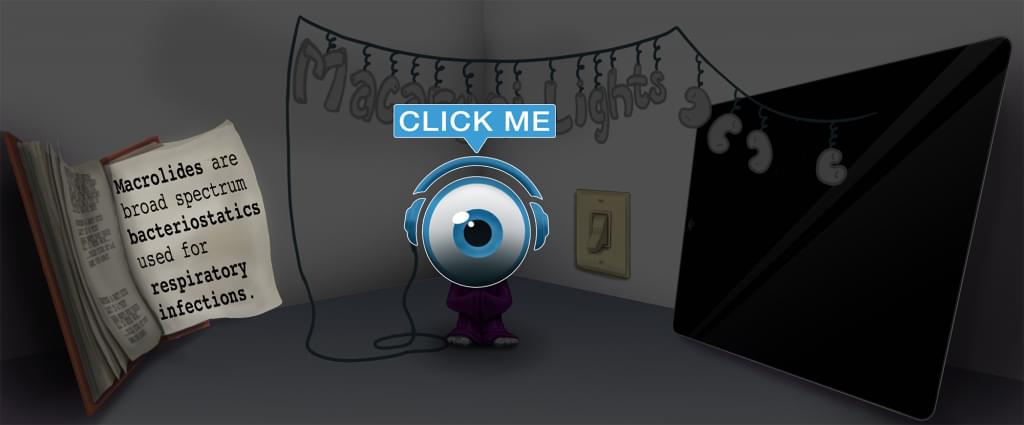# Master Ratio and Proportion with Picmonic for Nursing RN

## With Picmonic, facts become pictures. We've taken what the science shows - image mnemonics work - but we've boosted the effectiveness by building and associating memorable characters, interesting audio stories, and built-in quizzing.

### Ratio and ProportionPicmonic
The ratio and proportion method is one of the ways to solve dosage calculations. A ratio is two numbers separated by a colon (:). A colon indicates the need to divide. A proportion is two ratios of equal value. A proportion can be written as two fractions or ratios on either side of a double colon (::). A double colon and an equal sign are interchangeable. In a proportion equation, the first and last numbers are called the extremes, and the second and third numbers are the means. When setting up the proportion, you will need to put the ratios in the same arrangement. One of the ratios will contain an x indicating the unknown value. Next you will cross-multiply the means and extremes, and then divide both sides by the number before the x.
9 KEY FACTS
RATIO
Two Numbers Separated by a Colon
Two Numbers Separated by a Colon

A ratio indicates a connection between two numbers that is separated by a colon.

Colon Indicates Division
Colon Same as Division-sign

A colon signifies the use of division. A colon is interchangeable with a division sign.

PROPORTION
Equation with Two Equal Ratios
Potion is Filled with Two Equal Radios

A proportion is an equation that has two equal ratios. For example: 1/3 = 3/9.

Double Colons Indicates Equal Sign
Double Colon Same as Equal Sign

When there is a double colon between two ratios, it indicates an equivalent value between the ratios. A double colon is interchangeable with an equal sign.

Extremes
Extreme X

The extremes in a proportion are the first and last numbers. For example: in 1/3 = 3/9, 1 and 9 are the extremes.

Means
Mean M

The means are the second and third numbers in a proportion. For example: in 1/3 = 3/9, 3 and 3 are the means.

CALCULATING THE DOSE
Set up the Proportion
Set Up Potion

When setting up the proportion, you will need to put the ratio in the same arrangement. For example: mg/mL = mg/mL. When you are setting up the proportion remember that one of the ratios will contain an “x” because the amount is unknown. Example: 100mg/5mL = 160mg/xmL)

Cross-Multiply Means and Extremes
Cross-Multiply Mean M and Extreme X

The second part of the equation is to cross-multiply the means and extremes. Using the above proportion it would look like 100x = 5 x 160.

Divide Both Sides by the Number Before Unknown Variable
Divide Both Sides by the Number Before Question-mark

The final step to determine the dose is to divide both sides by the number before x. Example: 100x/100 = 800/100. This would give you x = 8mL.

Unlock all 9 facts & definitions with Picmonic Free!

JOIN FREE

## Take the Ratio and Proportion Quiz

Picmonic's rapid review multiple-choice quiz allows you to assess your knowledge.

START QUIZ NOW

## Our Story Mnemonics Increase Mastery and Retention

Memorize facts with phonetic mnemonics

Unforgettable characters with concise but impactful videos (2-4 min each)

### Ace Your Nursing (RN) Classes & Exams with Picmonic:

#### Over 930,000 students use Picmonic’s picture mnemonics to improve knowledge, retention, and exam performance.